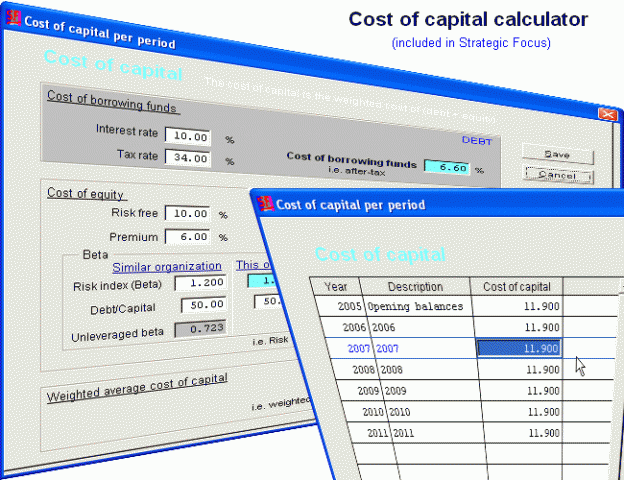Cost of Capital Calculator#### Cost of capital calculator in financial statement analysis

The cost of capital calculator provides a cost of capital per period which are generally annual, year to date, monthly or quarterly

The cost of capital is used to perform the financial analysis required by management. In business there is a ratio between the debt and equity of the entity. This debt equity ratio reflects the portion of borrowed funds vs equity and impacts on the business valuation.

There is a cost for borrowing funds which is influenced by the interest rate and tax rate prevalent at the time. The weighted average cost of capital takes into account both the cost of borrowed funds and the cost of equity.